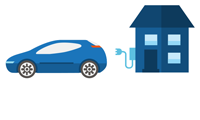## Calculate the consumption of your appliances and devices*

There are a dozen calculators for you to choose from. Learn more about the energy consumption of your appliances and electronics to make wiser energy choices.Since electricity costs so much less than gas, see what you could save when you drive electric!

* Please note that these calculations are approximate, since a number of factors can affect consumption, including the actual efficiency of your appliances, the hours of use and weather conditions.

### kWh: definition and calculation method

• The kilowatthour (kWh) is a unit that measures energy.
• One kWh is equal to the consumption of one 1,000-watt electronic device in one hour.

To calculate the consumption of a device, multiply its power by the number of hours of use. Then, divide the result by 1,000 to obtain the consumption in kWh.

Find out more about the kilowatthour

## Discover the main sources of household energy consumption.

The main sources of electricity use in your home may come as a surprise.